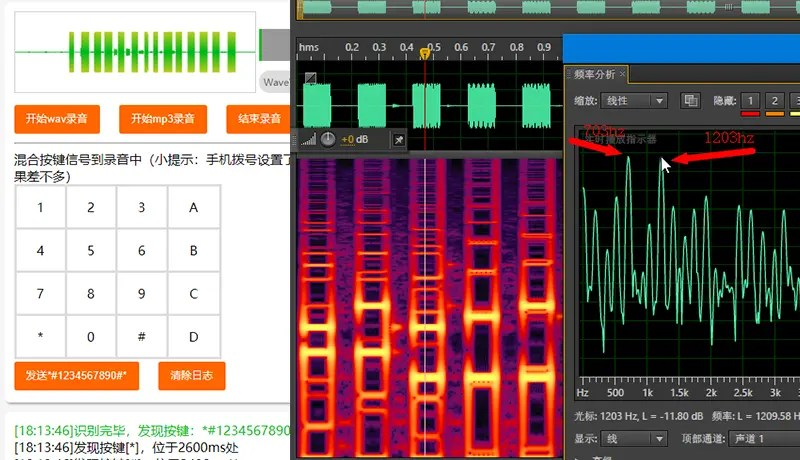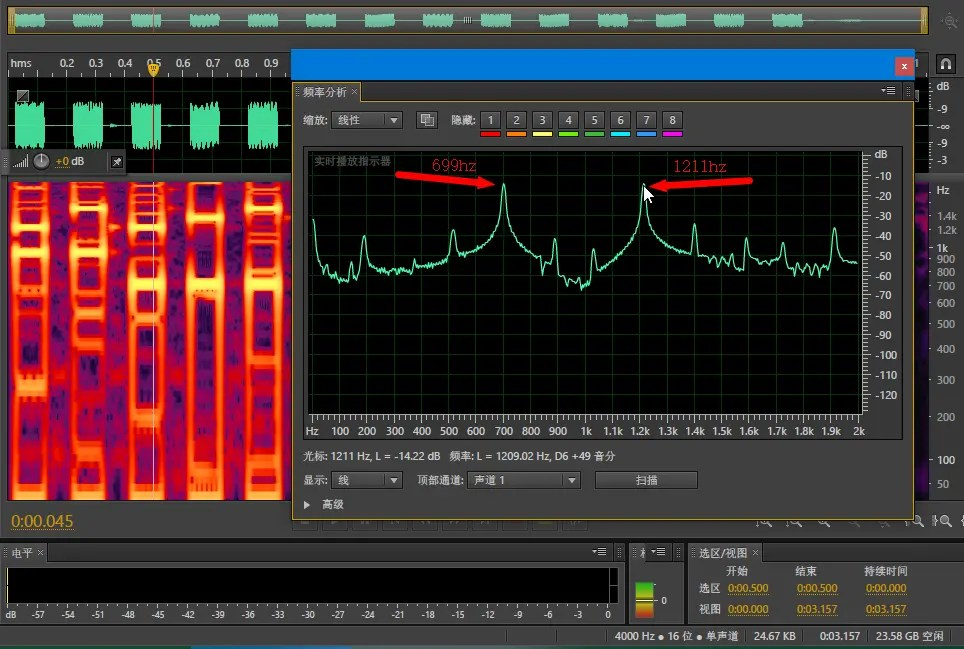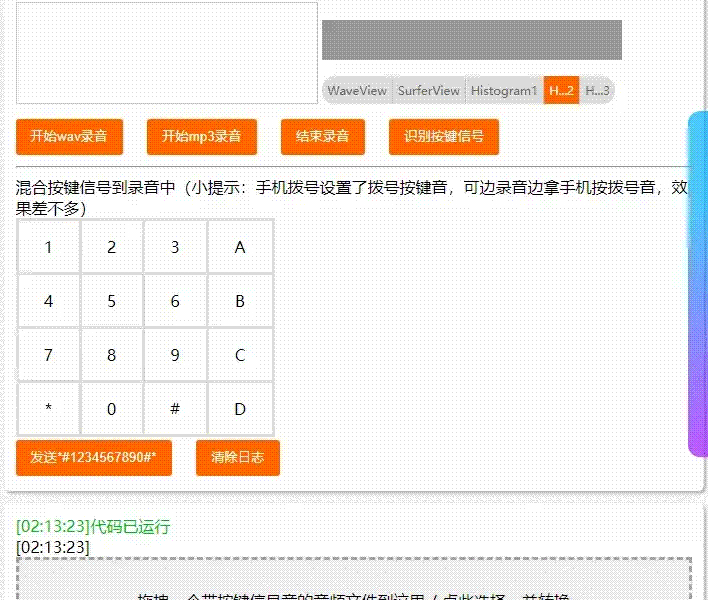# HTML5 implements DTMF (telephone dialing key signal) decoding and encoding, the code is simple and easy to transplant

Posted Jun 28, 20209 min read

DTMF(Dual Tone Multi Frequency) is composed of high frequency group and low frequency group. The high and low frequency groups each contain 4 frequencies; two frequency waveforms synthesize key signals(0-9 * # A B C D).

The method of detecting DTMF signal in SIP:SIPINFO, RFC2833, INBAND; As for what these are, my layman is purely lively; take two phones to call each other, and the sound of the button pressed halfway is to directly transmit DTMF through voice The signal belongs to INBAND(in-band detection).

Use Adobe Audition to open the phone recording file on the phone, you can visually see the neat DTMF signal, and analyze it to quickly get the decoding and encoding principles of this signal.

Online test address:[online test]( https://xiangyuecn.github.io/Recorder/assets/%E5%B7%A5%E5%85%B7-%E4%BB%A3%E7%A0%81%E8%BF%90%E8%A1%8C%E5%92%8C%E9%9D%99%E6%80%81%E5%88%86%E5%8F%91Runtime.html?jsname=teach.dtmf . decode_and_encode)[Figure 1]The simple and crude synthesis of PCM signal has more clutter, but it is similar to the recording signal produced by Huawei mobile phones(They have less clutter)

# I. Introduction

## 1.1 Some motivations for HTML5 to implement DTMF

My GitHub open source library Recorder is becoming more and more functional. Recently, some projects may use the DTMF decoding function, so I implemented it with js, which is easy to port. For the purpose, the relevant code is simple pure js code, and it is very convenient to transplant to other languages.

Three source codes are involved, all of which are small:

1. FFT: lib.fft.js 111 lines(code + blank line + comment)
2. DTMF decoding: dtmf.decode.js 192 lines(code + blank line + comment)
3. DTMF encoding: dtmf.encode.js 191 lines(code + blank line + comment)

Self-evaluation:High performance ?, high accuracy ?, low false recognition rate ?; welcome to online test , download another software dtmf2num(command line) Let's compare the damage.

## 1.2 Some effective scenarios

###(1) 10086

Please press 1 to check the call charge(you pressed a 1), the balance of your call charge is 990 million... It is undeniable that the realization of these capabilities is based on the encoding and decoding of DTMF signals.

###(2) Softphone

Through some channels, for example, the program on your server has the ability to automatically make calls. You want to implement some functions after the user presses certain keys, such as entering a password, so your server-side program needs to bring DTMF Decoding function.

###(3) Small toys

Write some small toys to play with. Hey ha?.

1. # DTMF frequency key comparison table

Low Frequency Group/High Frequency Group(hz) 1209 1336 1477 1633
697 1 2 3 A
770 4 5 6 B
852 7 8 9 C
941 * 0 # D
1. # Decode the DTMF signal to get the key value

## 3.1 Learn to decode manually

Observe the above [Figure 1], in a long PCM audio, two very bright horizontal lines can be clearly seen in the spectrum of each key signal(the signal energy corresponding to this frequency is very strong), and the need to locate it in Adobe Audition The time position of the analysis, and then click the menu:Window -> Frequency Analysis(Alt+Z), display the frequency information to get the two highest frequencies; the two highest frequencies are the frequency values in the frequency comparison table above(take the closest value):The low frequency 703hz is approximately equal to 697, and the high frequency 1203hz is approximately equal to 1209. Looking up the table, the key corresponding to this signal is "1".

## 3.2 understand some principles

Not professional, just look.

###(1) Adjusting the PCM sampling rate will basically not interfere with the DTMF signal

I said. Because the highest frequency of DTMF signal is 1633hz, which is far lower than the highest recognition frequency corresponding to the common sampling rates of `8000`(frequency up to 4000hz) and `44100`(frequency up to 22050hz).

###(2) Reducing the sampling rate is conducive to identifying DTMF signals

I said. For example:`8000` sampling rate contains 0-4000hz frequency signal, `44100` sampling rate contains 0-22050hz frequency signal, which is equivalent to `44100` more than `8000` 4000-22050hz and has nothing to do with DTMF signal The frequency, and it is accounted for. The most intuitive withdrawal of these extra frequencies is to increase the amount of calculation(exponential).

By analogy, if we control the highest frequency of PCM to be higher than 1633, it will greatly reduce the amount of calculation, such as limiting the maximum frequency of 2000hz, the corresponding sampling rate is `4000`, which is twice as small as `8000` , Cut off all high-frequency signals, refer to the following [Figure 2].

###(3) Putonghua is difficult to be just a DTMF signal

At least people say so. It happened that there was a sound that lasted for a while, and the highest two frequencies of this sound happened to be in the DTMF comparison table. The probability would not be too high.

Depending on the quality of the decoding algorithm, for the same audio, some decoders may mistakenly recognize 20 key signals, and some may only mistakenly recognize 2 key signals(such as the decoder I wrote, ha?).

## 3.3 Implement software decoding

The most intuitive implementation of soft decoding is to implement [2.1 manual decoding]in sequence and use a program. It is simple and crude, and does not require more principles and basic knowledge. Soft solution js source code: dtmf.decode.js

###(1) Reduce the sampling rate of PCM

In order to reduce the amount of calculation and highlight the frequency of the DTMF signal, we reduce the sampling rate of any PCM data to 4000, and the frequency of 0-2000hz is included in the PCM at this time. The simplest resampling method can be used:one data is extracted every few data; for example, the sampling rate of 16000 is reduced to 4000, and one can be taken every 4 samples. This processing performance cost is negligible.[Figure 2]Two frequencies are very prominent at 4000 sampling rate(Audition spectrum to the right of the right scale to decrease Resolution, otherwise the sampling rate of 4000 is a sloppy spectrum)

###(2) How to find the two horizontal lines

As shown in [Figure 2]above, there are two very strong frequencies(two very bright horizontal lines) in the frequency spectrum of a key signal, corresponding to the low frequency and high frequency of DTMF, these two frequencies will last for a period of time Therefore, as long as we find that there are two strongest frequencies in PCM, and these two frequencies are in the DTMF frequency table, then we can assume that there may be a DTMF key signal at this time position(note that there may be, not necessarily A key signal).

Then we only need to calculate whether there are two maximum frequency signals in a certain time period in the DTMF frequency table to achieve judgment; in addition to the use of FFT(fast Fourier transform), the calculation method is more commonly used Goertzel algorithm, Following the principle of entry to abandonment, we used a more general FFT to calculate the frequency, and Goertzel gave up learning.

It seems that the FFT operation will cause performance problems, but for short PCM calculations, it is also negligible, and we have reduced the sampling rate(the amount of calculation decreases exponentially); here is a data:a 4 minutes and 30 seconds The total time consumed by mp3 to perform a DTMF decoding is less than 300ms, and a total of about((4.5601000ms)/16ms = 16875` FFT calculations(where 16ms is the length of one sliding time in the sliding window below), fftSize=256.

###(3) Use FFT to convert time domain signal to frequency signal

FFT is a complicated thing, but fortunately there are many codes that can be copied. Reference js code: lib.fft.js

The FFT needs to provide a fftSize. The larger the frequency, the higher the resolution. For example, `fftSize=1024`, the resolution is:`4000/1024 = 3.90625hz`(4000 is the sampling rate of PCM). After FFT calculation, an array of `Int` will be output. The frequency of the first point in the array is `1 * 3.90625 = 3.90625 hz`, and the frequency of the last point is `512 * 3.90625 = 2000 hz`; in the array Each value of is the signal strength value of the corresponding frequency(convertible to decibels), the larger the signal, the stronger the signal.

But this resolution is not the bigger the better, because the larger the fftSize you provide, you need to provide the same amount of PCM sampling data for each calculation, `fftSize=1024` will provide the PCM data of `1024/4000*1000 = 256ms` This problem arises:the duration of our single DTMF tone may be 40-100 ms, and the data interval covered by 256ms is too long or may even be covered by two key signals; therefore, we need to lower the resolution rate.

The compromise result after lowering is:`fftSize=256`, the resolution is `4000/256 = 15.625 hz`(reduced by 4 times compared to 3.90625hz resolution), it can t be lower, it will be recognized at the lower resolution It is not clear which value in the DTMF frequency table the signal is. At this time, the length of PCM data required for each calculation is `256/4000*1000 = 64ms`, which can ensure that there is only one key signal in the interval.

###(4) Rough FFT sweep mode:sliding window without letting go of any possible signal

We can't simply divide the PCM into N segments(256 samples into one segment), and then perform an FFT calculation for each segment, which will split a signal into two segments of data with high probability, resulting in the detection of this signal. Therefore, we should use the sliding window mode when calculating the FFT, sliding the calculation window forward a little bit each time, so as to ensure that all data can be calculated at least once.

You can set the size of each slide to 1/4 of the window size, that is, 256 samples are the window size, and slide forward 256/4 = 64 samples per FFT calculation(64/4000*1000 = 16 ms `), so that all signals can be perfectly covered, see below [Figure 3].[Figure 3]The following non-stop sliding window can cover all signal areas very well, the disadvantage is one calculation It needs to be calculated 4 times; although the above kind only needs one calculation, the coverage is too poor

###(5) The same signal that appears continuously is the valid key

A signal that appears only once does not mean that it is a valid DTMF key signal. The same signal that appears three times in a row is determined to be a valid signal. Therefore, the minimum key tone duration we can recognize is:`256/4000*1000 = 64ms`, `64/4 = 16 ms`, `16 *(3-0.999999?) 32 ms`. There is no limit to the length of the longer button sound, because the same consecutive ones will only count as one button signal.

In addition, we need to distinguish the gap between the two buttons. We define that there are more than 3 areas where there is no signal. The next signal is the new button signal, so that the same button can be distinguished multiple times. Therefore, the two signals theory The minimum interval time on the upper is:`16 * 3 + 16 * 3 = 96 ms`, but the actual calculation result is the minimum boundary 3 times, and the fault tolerance is better if it is more than 3+1 times. The optimal interval should be `16 * 4 + 16 * 4 = 128 ms or more, which means that after pressing a key, the next key will be pressed after 128 ms(generating a signal).

Keep calculating backwards, until the end of PCM, we can find out all the DTMF signals, and we can more accurately convert the position of these signals. Then test it:high accuracy, low false recognition rate, good performance, and good results(promotion and salary increase).

1. # DTMF signal encoding Generate key PCM audio signal

Not professional, just look. With the foundation of decoding, it is easy to write the signal generation code. We only need to generate the two frequency waveforms, and then merge them together, and then put multiple signals into the PCM at a certain interval; the actual code is written according to this set of logic, and the signal encoding js source code: dtmf.encode.js

## 4.1 Mix:mixing of two audio signals

Whether it is generating a single key signal or mixing the key signal into the voice PCM stream, the operation of signal mixing is involved. It seems to be a deep thing; do IFFT calculations? No matter how complicated it is, let's try it with a simple mixing algorithm:`c =(a+b)/2` is so simple and rude, but this linear averaged sound has a lot of noise.

Finally, using `c = a + b-(a * b/±0x7FFF)`, the sound quality after mixing is very good, from this article Article, the final source code read the `Mix` function in dtmf.encode.js above.

## 4.2 Generate a single key signal

The source code reads the `Recorder.DTMF_Encode` function in dtmf.encode.js above. For example, to generate the signal of the "1" key, look up the table to get a low frequency `697 hz` and a high frequency `1209 hz`, and then generate a sine wave PCM signal of two frequencies separately, and mix the two PCM with the `Mix` function You can get the signal of the "1" key.

The generated code is also surprisingly simple, but it is limited by the simple mixing algorithm used by the `Mix` function. There are a lot of clutter after the two frequency sine waves are superimposed. See above [Figure 1]the two largest frequencies. The wave signal is also very strong, but fortunately it does not affect the recognition.

## 4.3 Multiple consecutive key signals are mixed into the voice PCM stream

This is the actual and practical function:`EncodeMix.prototype.mix(pcms, sampleRate, index)` in dtmf.encode.js above, no matter how many buttons you press at once, the mixing function will be step by step one by one Mixed into the voice stream, and ensure that the interval between keys can be correctly recognized by the decoding program.

This code is relatively simple, and it does two things in total:delay + call the `Mix` function, where the Mix call actually replaces the PCM and not two PCM mixes.

Finally, let's finish with a moving picture:= end =